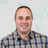Foundation

## Foundation Forum

##### Add a fixed number to equalizer height
• 2
Replies

Hello,

Is it possible to add a fixed number to the height calculated by equalizer?

So for example if the height of the columns calculated from equalizer is 350px, I would like to add 35px to that so the final height that will be applied is height: 385px instead of height: 350px.

Thank you,
-R

• 2
Replies

Hello,

Is it possible to add a fixed number to the height calculated by equalizer?

So for example if the height of the columns calculated from equalizer is 350px, I would like to add 35px to that so the final height that will be applied is height: 385px instead of height: 350px.

Thank you,
-R

This post has been closed. No new replies can be added.

#####Rafi Benkualalmost 4 years ago

You can add padding to the container you are equalizing or padding to the elements inside to make the box taller - http://codepen.io/rafibomb/pen/gaoYmg

#####Rennyalmost 4 years ago

For those interested in the answer to this. Here is the solution:

``` \$(document).foundation({
equalizer: {
after_height_change: function(){
\$('div[data-equalizer-watch]').height(function (index, height) {
return (height + 35);
});
}
}
});
```